Courses

# The Reaction Coordinate Civil Engineering (CE) Notes | EduRev

## Civil Engineering (CE) : The Reaction Coordinate Civil Engineering (CE) Notes | EduRev

The document The Reaction Coordinate Civil Engineering (CE) Notes | EduRev is a part of the Civil Engineering (CE) Course Thermodynamics.
All you need of Civil Engineering (CE) at this link: Civil Engineering (CE)

The Reaction Coordinate

Consider again the general chemical reaction depicted in eqn. 8.1: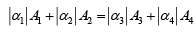During the progress of the reaction, at each point the extent of depletion of the reactants, and the enhancement in the amount of product is exactly in proportion to their respective stoichiometric coefficients. Thus for any change dn i in the number of moles of the ith progress of the reaction one may write: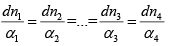..(8.21)

Since all terms are equal, they can all be set equal to a single quantity dξ , defined to represent the extent of reaction as follows: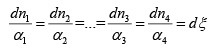..(8.22)

The general relation between a differential change dni din the number of moles of a reacting species and dξ is therefore: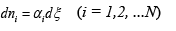..(8.23)

This new variable ξ , called the reaction coordinate, describe the extent of conversion of reactants to products for a reaction. Thus, it follows that the value of ξ is zero at the start of the reaction. On the other hand whenξ = 1 , it follows that the reaction has progressed to an extent at which point each reactant has depleted by an amount equal to its stoichiometric number of moles while each product has formed also in an amount equal to its stoichiometric number of moles. For dimensional consistency one designates such a degree of reaction as corresponding to ∆ξ= 1 mole.

Now, considering that at the point where the reaction has proceeded to an arbitrary extent characterized by ξ (such thatξ > 0 ), the number of moles of ith species is nwe obtain the following relation: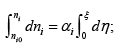where η, is a dummy variable and nio = initial number of moles of  'i' Thus: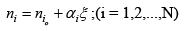...(8.24)

Thus the total number of moles of all species corresponding toξ extent of reaction: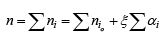...(8.25)

Or: n =n0 + αξ                        ...(8.26)

Where: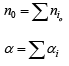...(8.27 & 8.28)

Thus;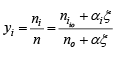...(8.29)

The foregoing approach may be easily extended to develop the corresponding relations for a set of multiple, independent reactions which may occur in a thermodynamic system. In such a case each reaction is assigned an autonomous reaction co-ordinate ξ j (to represent the jth reaction). Further the stoichiometric coefficient of the ith species as it appears in the jth reaction is designated by α i , j . Since a species may participate in more than a single reaction, the change in the total number of moles of the species at any point of time would be the sum of the change due each independent reaction; thus, in general: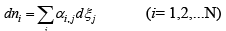...(8.30)

On integrating the above equation starting from the initial number of moles nio to ni corresponding to the reaction coordinate ξ j of each reaction: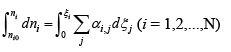...(8.31)

Or: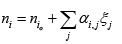...(8.32)

Summing over all species gives: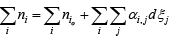...(8.33)

Now: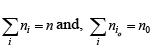...(8.34)

We may interchange the order of the summation on the right side of eqn. (8.33); thus: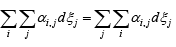...(8.35)

Thus, using eqns. 8.34 and 8.35, eqn. 8.33 may be written as: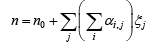...(8.36)
In the same manner as eqn. 8.28, one may write: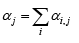...(8.37)

Thus eqn. 8.33 becomes: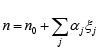...(8.38)

Using eqns. 8.32 and 8.38 one finally obtains: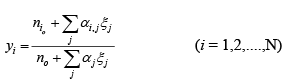...(8.39)

Offer running on EduRev: Apply code STAYHOME200 to get INR 200 off on our premium plan EduRev Infinity!

## Thermodynamics

56 videos|92 docs|33 tests

,

,

,

,

,

,

,

,

,

,

,

,

,

,

,

,

,

,

,

,

,

;